# Exceptional value

(diff) ← Older revision | Latest revision (diff) | Newer revision → (diff)

A concept in value-distribution theory. Letbe a meromorphic function in the whole-plane and let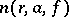denote its number of-points (counting multiplicities) in the disc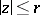. According to R. Nevanlinna's first fundamental theorem (cf. ), as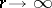,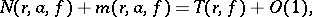where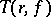is the characteristic function, which does not depend on,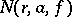is the counting function (the logarithmic average of) andis a function expressing the average proximity of the values oftoon(cf. Value-distribution theory). For the majority of valuesthe quantitiesandare asymptotically equivalent, as. A (finite or infinite) numberis called an exceptional value if this equivalence asis violated. One distinguishes several kinds of exceptional values.

A numberis called an exceptional value ofin the sense of Poincaré if the number of-points ofin the whole plane is finite (cf. , ), in particular if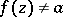for any.

A numberis called an exceptional value ofin the sense of Borel ifgrows slower, in a certain sense, than, as(cf. , ).

A numberis called an exceptional value ofin the sense of Nevanlinna (cf. ) if its defect (cf. Defective value)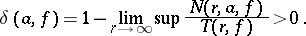A numberis called an exceptional value ofin the sense of Valiron if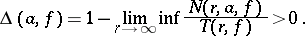A numberfor which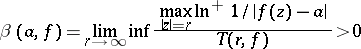is also called an exceptional value for. The quantity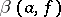(the positive deviation of) characterizes the rate of the asymptotic approximation ofto(cf. ).

How to Cite This Entry:
Exceptional value. Encyclopedia of Mathematics. URL: http://encyclopediaofmath.org/index.php?title=Exceptional_value&oldid=18994
This article was adapted from an original article by V.P. Petrenko (originator), which appeared in Encyclopedia of Mathematics - ISBN 1402006098. See original article